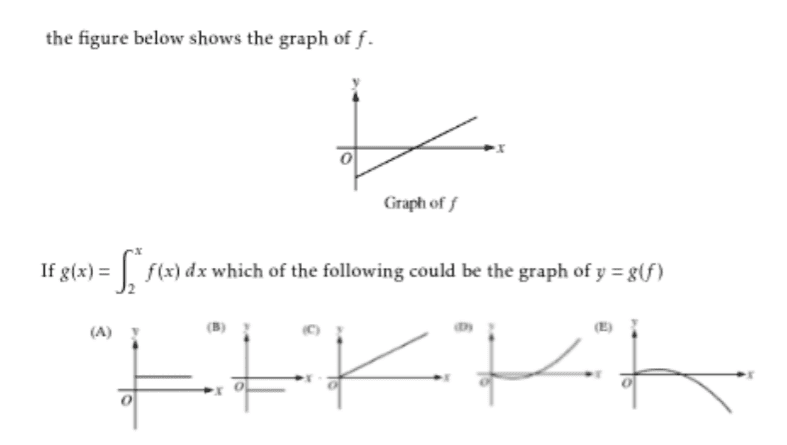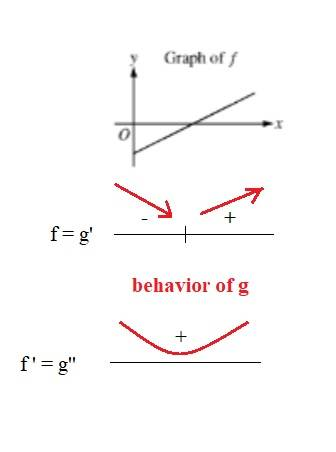# -apc.4.2.9 graph of f(x) to g(x)

• MHB
Gold Member
MHBimage due to graphs

ok just by observation I chose D since integrals tend to introduce curves not eliminate them and the slope was positive

skeeter
two significant typos in this problem ...

(1) should be $\displaystyle g(x) = \int_2^x f(t) \, dt$,

(2) which of the following could be $y=g(x)$, not $g(f)$

$g’(x) = f(x) \implies$ the graph of $f(x)$ is the graph of the derivative of $g(x)$

so ... what do you think?

Gold Member
MHB
good catch

HOI
https://www.physicsforums.com/attachments/10736
image due to graphs

ok just by observation I chose D since integrals tend to introduce curves not eliminate them and the slope was positive
You should be able to say much more than that! Since the graph of f is a straight line, f is "linear" and its integral is quadratic so its graph is a parabola. Further since the graph of f is below the x-axis the integral starts out negative and becomes positive. Yes, the slope of f is positive so the curvature of g is positive. THAT is why "D" is the correct choice!

skeeterGold Member
MHB
graphs always help a lot

Gold Member
MHB
Here is a WIP of a PDF of the MHB replies to the AP Calculus problems given here
A counter has been put into the overleaf document which at this posting is 40,000 views of the replies
My goal is to make the PDF error free and reach 100 problems
Mahalo
https://dl.orangedox.com/6rStfn4eMFHuHvAKuX

Last edited:
HOI
"WIP', "PDF", "AP"? I thought we were supposed to use English on this site!

Gold Member
MHB
WIP Work In Progress

Last edited: